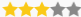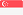# Hashing Definition - What is Hashing?

A hash is the result of a mathematical calculation. It converts an amount of data to a smaller value, the hash. For example, you could convert a long password into a short string. This only works in one direction, so you cannot convert a hash back to the original amount of data. With crypto currencies (BitcoinLitecoinDogecoin etc.) such calculations are done to find the next block in the blockchain.

So all miners are calculating and whoever finds the right solution first gets the reward. The faster the PC or graphics card, the more hashes are calculated per second. Since it is almost impossible to find the right solution first, many miners join together to form a mining pool.

## Crypto Glossary / Lexicon